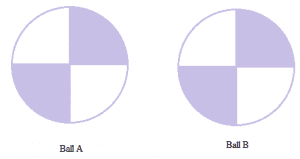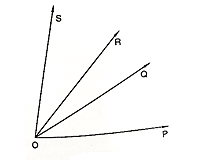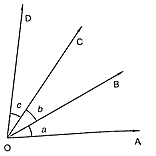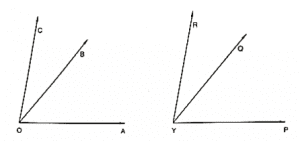×#### Thank you for registering.

One of our academic counsellors will contact you within 1 working day.

Click to Chat

1800-1023-196

+91-120-4616500

CART 0

• 0

MY CART (5)

Use Coupon: CART20 and get 20% off on all online Study Material

ITEM
DETAILS
MRP
DISCOUNT
FINAL PRICE
Total Price: Rs.

There are no items in this cart.
Continue Shopping• Complete JEE Main/Advanced Course and Test Series
• OFFERED PRICE: Rs. 15,900
• View Details

Congruence Exercise 16.1

Question: 1

Explain the concept of congruence of figures with the help of certain examples.

Solution:

Congruent objects or figures are exact copies of each other or we can say mirror images of each other. The relation of two objects being congruent is called congruence.

Consider Ball A and Ball B. These two balls are congruent.Now consider the two stars below. Star A and Star B are exactly the same in size, colour and shape. These are congruent stars.Question: 2

Fill in the blanks:

(i) Two line segments are congruent if ___

(ii) Two angles are congruent if ___

(iii) Two square are congruent if ___

(iv) Two rectangles are congruent if ___

(v) Two circles are congruent if ___

Solution:

(i) They have the same length, since they can superpose on each other.

(ii) Their measures are the same. On superposition, we can see that the angles are equal.

(iii) Their sides are equal. All the sides of a square are equal and if two squares have equal sides, then all their sides are of the same length. Also angles of a square are 90° which is also the same for both the squares.

(iv) Their lengths are equal and their breadths are also equal. The opposite sides of a rectangle are equal. So if two rectangles have lengths of the same size and breadths of the same size, then they are congruent to each other.

(v) Their radii are of the same length. Then the circles will have the same diameter and thus will be congruent to each other.

Question: 3

In Figure, ∠POQ ≅ ∠ROS, can we say that ∠POR ≅ ∠QOSSolution:

We have,

∠POQ ≅ ∠ROS (1) Also, ∠ROQ ≅ ∠ROQ Therefore adding ∠ROQ to both sides of (1), Weget, ∠POQ + ∠ROQ ≅ ∠ROQ + ∠ROS Therefore, ∠PQR = ∠QOS

Question: 4

In figure, a = b = c, name the angle which is congruent to ∠AOC

Solution:We have,

∠ AOB = ∠ BOC = ∠ COD

Therefore, ∠ AOB = ∠ COD

Also, ∠ AOB + ∠ BOC = ∠ BOC + ∠ COD

∠ AOC = ∠ BOD

Hence, ∠ BOD is congruent to ∠ AOC

Question: 5

Is it correct to say that any two right angles are congruent? Give reasons to justify your answer.

Solution:

Two right angles are congruent to each other because they both measure 90 degrees.

We know that two angles are congruent if they have the same measure.

Question: 6

In figure, ∠AOC ≅ ∠PYR and ∠BOC ≅ ∠QYR. Name the angle which is congruent to ∠AOB.Solution:

∠AOC ≅ ∠PYR…. (i) Also, ∠BOC ≅ ∠QYR Now, ∠AOC = ∠AOB + ∠BOC ∠PYR = ∠PYQ +∠QYR By putting the value of ∠AOC and ∠PYR in equation (i), we get, ∠AOB + ∠BOC ≅ ∠PYQ + ∠QYR ∠AOB ≅ ∠PYQ (∠BOC ≅ ∠QYR) Hence, ∠AOB ≅ ∠PYQ

Question: 7

Which of the following statements are true and which are false;

(i) All squares are congruent.

(ii) If two squares have equal areas, they are congruent.

(iii) If two rectangles have equal areas, they are congruent.

(iv) If two triangles have equal areas, they are congruent.

Solution:

(i) False.

All the sides of a square are of equal length.

However, different squares can have sides of different lengths. Hence all squares are not congruent.

(ii) True.

Area of a square = side x side

Therefore, two squares that have the same area will have sides of the same lengths. Hence they will be congruent.

(iii) False Area of a rectangle = length x breadth

Two rectangles can have the same area. However, the lengths of their sides can vary and hence they are not congruent.

Example: Suppose rectangle 1 has sides 8 m and 8 m and area 64 meter square. Rectangle 2 has sides 16 m and 4 m and area 64 meter square. Then rectangle 1 and 2 are not congruent.

(iv) False

Area of a triangle = 12 x base x height

Two triangles can have the same area but the lengths of their sides can vary and hence they cannot be congruent.### Course Features

• 728 Video Lectures
• Revision Notes
• Previous Year Papers
• Mind Map
• Study Planner
• NCERT Solutions
• Discussion Forum
• Test paper with Video Solution